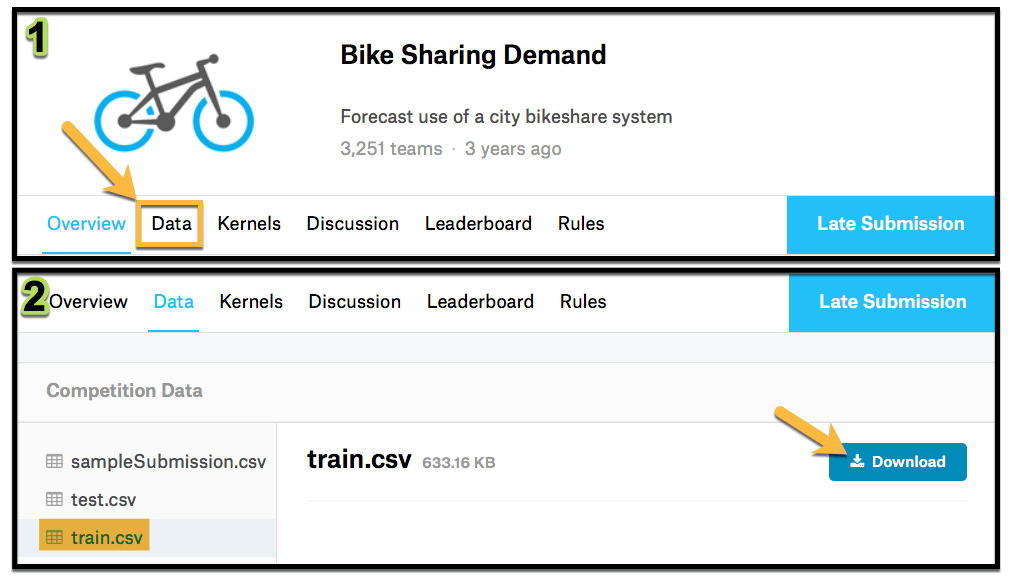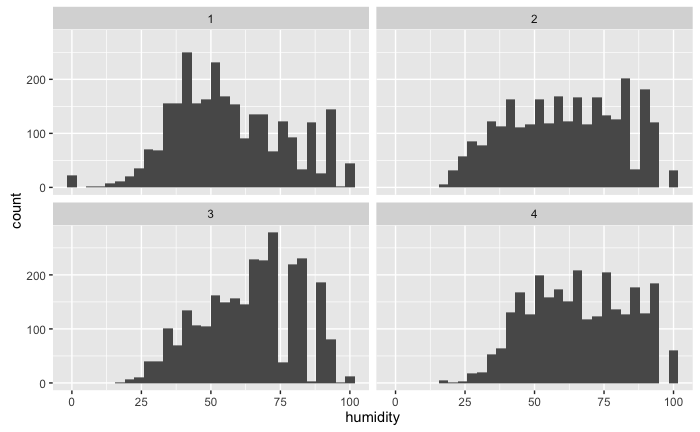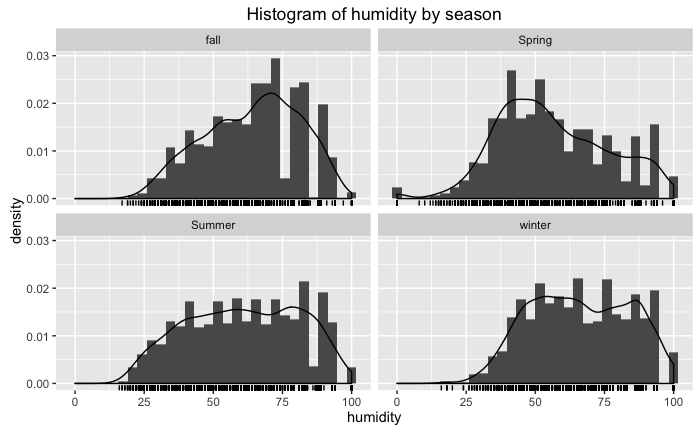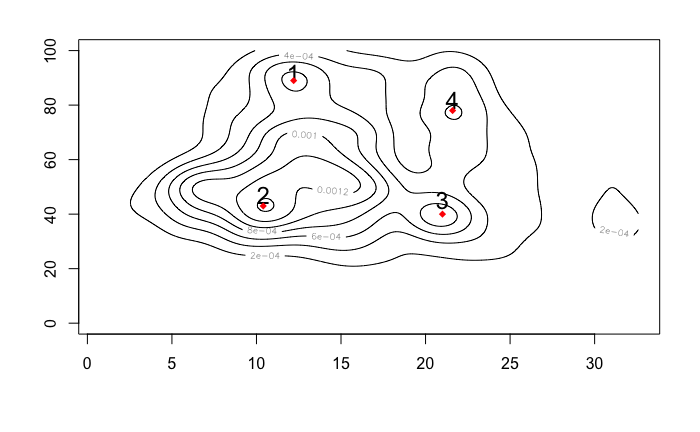Tutorials
r programming

Bivariate Distribution Heatmaps in R

Learn how to visually show the relationship between two features, how they interact with each other, and where data points are concentrated.

As a data scientist, you will have to analyze the distribution of the features in your dataset. Usually, this is done by using histograms, this is really useful to show the variable range of values, their deviation and where values are concentrated.

Tutorial objectives

In this tutorial, you will learn how to

This tutorial uses R. If you're not yet familiar with R, I suggest you take our free Introduction to R course on DataCamp.

Bike Sharing Dataset

For this tutorial, you are going to use the Bike Sharing Demand Dataset from a kaggle competition.library(dplyr)
na.strings = FALSE,
strip.white = TRUE)
glimpse(bike)

Dataset Features

Feature Description
datetime hourly date + timestamp
season 1 = spring
2 = summer
3 = fall
4 = winter
holiday whether the day is considered a holiday
workingday whether the day is neither a weekend nor holiday
weather 1: Clear, Few clouds, Partly cloudy, Partly cloudy
2: Mist + Cloudy, Mist + Broken clouds, Mist + Few clouds, Mist
3: Light Snow, Light Rain + Thunderstorm + Scattered clouds, Light Rain + Scattered clouds
4: Heavy Rain + Ice Pallets + Thunderstorm + Mist, Snow + Fog
temp temperature in Celsius
atemp "feels like" temperature in Celsius
humidity relative humidity
windspeed wind speed
casual number of non-registered user rentals initiated
registered number of registered user rentals initiated
count number of total rentals

Feature Selection

You are going to analyze the relationship between atemp and humidity by season, so select them from the dataset. Remember to load dplyr package.

bike_data<-
bike %>%
dplyr::select(season, atemp, humidity)

Histograms

Ok now you are ready to start analyzing the data, start by creating a histogram for each of the features by season. You are going to do this using ggplot2 library so don´t forget to load it.

Humidity Histogram

library(ggplot2)
bike_data %>%
ggplot( aes(x=humidity) ) +
geom_histogram(bins=30) +
facet_wrap(~season,ncol = 2)With the histogram, you can see that there is a higher humidity during winter (which is obvious) but you can have a sense of how it is distributed during each season.

Now add the density, a density rug and change the names of the facets, just to have a better-looking visualization.

bike_data %>%
mutate(season_label = case_when(
season == 1 ~ "Spring",
season == 2 ~ "Summer",
season == 3 ~ "fall",
season == 4 ~ "winter")) %>%
ggplot( aes(x=humidity) ) +
geom_histogram(bins=30,aes(y = ..density..)) +
geom_rug()+
geom_density()+
facet_wrap(~season_label,ncol = 2)+
ggtitle("Histogram of humidity by season")Now, this gives you a better feel of how humidity behaves, do the same with atemp.

atemp Histogram

Reuse the above code and change the variable to atemp,

bike_data %>%
mutate(season_label = case_when(
season == 1 ~ "Spring",
season == 2 ~ "Summer",
season == 3 ~ "fall",
season == 4 ~ "winter")) %>%
ggplot( aes(x=atemp) ) +
geom_histogram(bins=30,aes(y = ..density..)) +
geom_rug()+
geom_density()+
facet_wrap(~season_label,ncol = 2)+
ggtitle("Histogram of atemp by season")

Histogram of atemp by seasonOk, so far so good, everything is going according to what you would expect for each season. In each graph, you have an approximation of the probability distribution function.

But it is difficult based on this graphs to visualize how the features interact with each other. The first difficulty you encounter is that your histogram needs to be in 3D because you're trying to find the distribution function for the two features.

Fitting a bivariate distribution to your data

The goal is to visualize the bivariate distribution, to be able to do this you first need to fit a bivariate distribution to the data. This will be done using the MASS library and the kde2d function. The kde2d function will estimate the bivariate distribution, assuming normality for the random variables. The inputs to this function are as follows,

input description
x x coordinate data
y y coordinate data
n number of grid point for each axis
lims limits for x and y

Now apply it to the dataset filtering for summer,

library(MASS)
bike_data_summer <- bike_data %>% filter(season==1)
bike_density <- kde2d(bike_data_summer$atemp,bike_data_summer$humidity, n=1000)

Note: When loading the MASS library it comes with a select function, this will have an issue with dplyr select function so to fix this when using the dplyr select you need to add dplyr:: to it like this dplyr::select() for it to work correctly.

Now look at the class and the structure of bike_density

class(bike_density)
str(bike_density)

It is a list that has 3 values x, y, and z. The first two have the features values and the third one is a matrix with the value of the pdf. Take a look at the density using the contour function,

contour(bike_density)
text(12.2,92,"1",cex=1.5)
points(12.2,89,col="red",pch=18)
text(10.4,47,"2",cex=1.5)
points(10.4,43,col="red",pch=18)
text(21,45,"3",cex=1.5)
points(21,40,col="red",pch=18)
text(21.6,82,"4",cex=1.5)
points(21.6,78,col="red",pch=18)Looking at this you can identify some accumulation points, that could be understood as the most frequent values for the combination of the two features. The values for each point are resumed on the following table,

point atemp (°C) hummidity (%)
1 12.2 89
2 10.4 43
3 21 43
4 21.6 78

Heatmap

To create your heatmap you first need to define the color scale you want to use, you can do this using the function colorRampPalette

hm_col_scale<-colorRampPalette(c("black","blue","green","orange","red"))(1000)

hm_col_scale is a vector going from black to red using RGB code in 1000 steps.

Now plot your heat map using the image function, this function is used to plot matrixes.

image(bike_density$z, col = hm_col_scale, zlim=c(min(bike_density$z), max(bike_density$z))) text(0.31,0.46,"Most Frequent",col="blue",cex=0.8) points(0.31,0.45,col="blue",pch=18)Now, as you can see this has more information than the contour plot because now the colors tell us how high the accumulation point goes. You can see that the most frequent values for humidity and atemp during spring is around the point$(10.4,43)\$

Conclusion

Now you know how to create a heat map, it's your turn to create your own. Start by looking at the other season and the relation with the other variables.

Think about how could you use this to gain insight on how many bikes you have to supply for the different values of the variables based on the fact that most frequent points have a higher probability.

If you would like to learn more about generating data visualizations with ggplot, be sure to take a look at our Data Visualization with ggplot2 course.